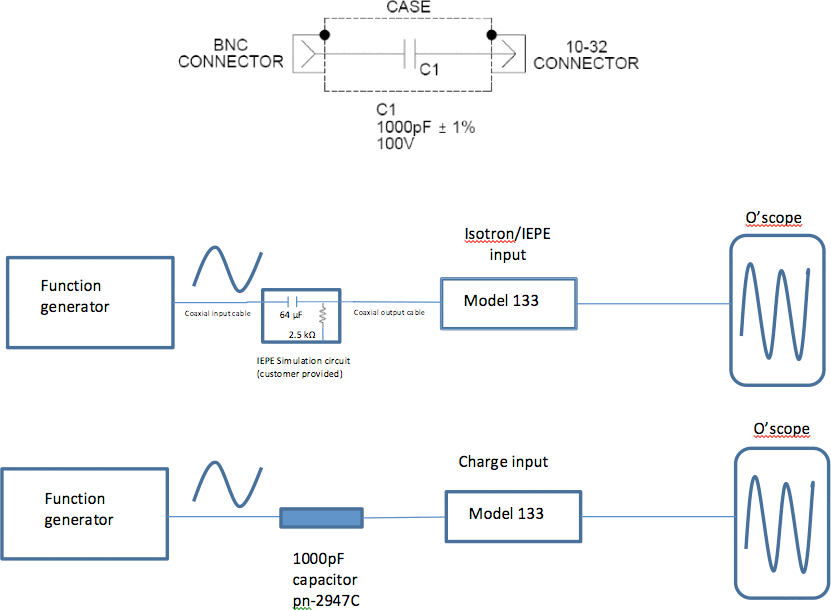Home/Resources/Ask The Experts/Calibrating the Model 133 via calibration coefficients

# Calibrating the Model 133 via calibration coefficients

### Question

What is the process for performing a calibration in-house with my Model 133 (3-channel) signal conditioner?

The calibration process is a "Special" mode function and is initiated by pressing the < Select Function > push button while simultaneously pressing the < Left > and < Right > EDITS push buttons. Refer to page 17 of IM133.

The calibration process will utilize a fixed input voltage specified in the instruction manual, to the appropriate input. For the charge inputs, the voltage applied will be through a precision 1000pF capacitor. See Fig. 1.

1. There are seven key main coefficients (K1-K7) *

• K1-K3 voltage input(Isotron input)

• K4 & K7(charge input)via, 1000pF capacitor

(1000pF capacitor- Endevco Sensing Systems p/n 2947C)Figure 1

• The transfer function is: Q = EC

• Where:
• Q = Charge, in picocoulombs (pC)

• E = Voltage, in volts (V)

• C = Capacitance, in picofarads (pF)

2. Gain Adjust- Fixed input voltage specified(at a frequency of 300Hz)

• K1-K3 (voltage applied to Isotron input), K4 & K7 (charge signal applied to charge input)

• Set required input signal for each coefficient;

• K1-30mV rms

• K2-300mV rms

• K3-3000 mV rms

• K4 & K7- 3000pC rms[ 3000mV rms into 1000pF capacitor = 3000pC rms]

• Adjust coefficients by < EDIT> button, i.e., adjusting calibration constant displayed on LED display until desired output is obtained.Figure 2

3. Verification of correct gain setting